18 Oct 2020

# EXTENDING PYTORCH - (Copied)

see: https://pytorch.org/docs/stable/notes/extending.html

In this note we’ll cover ways of extending torch.nn, torch.autograd, torch, and writing custom C extensions utilizing our C libraries.

## Extending torch.autograd

Adding operations to autograd requires implementing a new Function subclass for each operation. Recall that Functions are what autograd uses to compute the results and gradients, and encode the operation history. Every new function requires you to implement 2 methods:

• forward() - the code that performs the operation. It can take as many arguments as you want, with some of them being optional, if you specify the default values. All kinds of Python objects are accepted here. Tensor arguments that track history (i.e., with requires_grad=True) will be converted to ones that don’t track history before the call, and their use will be registered in the graph. Note that this logic won’t traverse lists/dicts/any other data structures and will only consider Tensors that are direct arguments to the call. You can return either a single Tensor output, or a tuple of Tensors if there are multiple outputs. Also, please refer to the docs of Function to find descriptions of useful methods that can be called only from forward().

• backward() - gradient formula. It will be given as many Tensor arguments as there were outputs, with each of them representing gradient w.r.t. that output. It should return as many Tensors as there were inputs, with each of them containing the gradient w.r.t. its corresponding input. If your inputs didn’t require gradient (needs_input_grad is a tuple of booleans indicating whether each input needs gradient computation), or were non-Tensor objects, you can return None. Also, if you have optional arguments to forward() you can return more gradients than there were inputs, as long as they’re all None.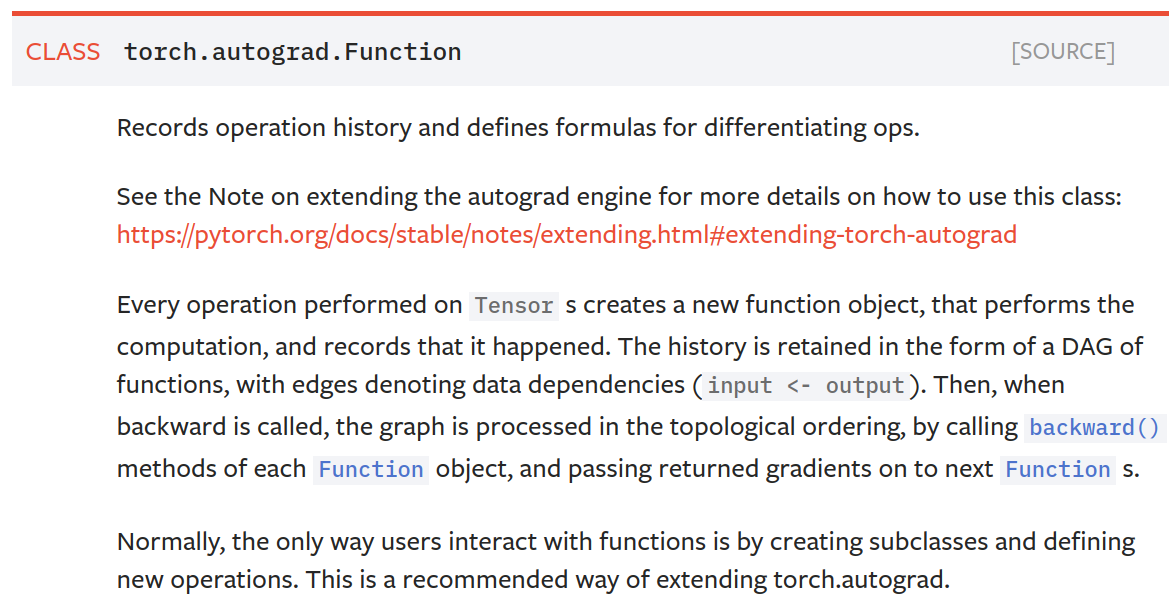### NOTE 1

It’s the user’s responsibility to use the special functions in the forward’s ctx properly in order to ensure that the new Function works properly with the autograd engine.

• save_for_backward() must be used when saving input or output of the forward to be used later in the backward.

• mark_dirty() must be used to mark any input that is modified inplace by the forward function.

• mark_non_differentiable() must be used to tell the engine if an output is not differentiable.

### NOTE 2

By default, all the output Tensors that are of differentiable type will be set to require gradient and have all autograd metadata set for them. If you don’t want them to require gradients, you can use the mark_non_differentiable method mentioned above. For output Tensors that are not of differentiable type (integer types for example), they won’t be marked as requiring gradients.

Below you can find code for a Linear function from torch.nn, with additional comments:

# Inherit from Function
class LinearFunction(Function):

# Note that both forward and backward are @staticmethods
@staticmethod
# bias is an optional argument
def forward(ctx, input, weight, bias=None):
ctx.save_for_backward(input, weight, bias)
output = input.mm(weight.t())
if bias is not None:
output += bias.unsqueeze(0).expand_as(output)
return output

# This function has only a single output, so it gets only one gradient
@staticmethod
def backward(ctx, grad_output):
# This is a pattern that is very convenient - at the top of backward
# unpack saved_tensors and initialize all gradients w.r.t. inputs to
# None. Thanks to the fact that additional trailing Nones are
# ignored, the return statement is simple even when the function has
# optional inputs.
input, weight, bias = ctx.saved_tensors
grad_input = grad_weight = grad_bias = None

# These needs_input_grad checks are optional and there only to
# improve efficiency. If you want to make your code simpler, you can
# skip them. Returning gradients for inputs that don't require it is
# not an error.
if ctx.needs_input_grad:
grad_input = grad_output.mm(weight)
if ctx.needs_input_grad:
grad_weight = grad_output.t().mm(input)
if bias is not None and ctx.needs_input_grad:
grad_bias = grad_output.sum(0)

return grad_input, grad_weight, grad_bias


Now, to make it easier to use these custom ops, we recommend aliasing their apply method:

linear = LinearFunction.apply

Here, we give an additional example of a function that is parametrized by non-Tensor arguments:

class MulConstant(Function):
@staticmethod
def forward(ctx, tensor, constant):
# ctx is a context object that can be used to stash information
# for backward computation
ctx.constant = constant
return tensor * constant

@staticmethod
def backward(ctx, grad_output):
# We return as many input gradients as there were arguments.
# Gradients of non-Tensor arguments to forward must be None.
return grad_output * ctx.constant, None


### NOTE 3

Inputs to backward, i.e., grad_output, can also be Tensors that track history. So if backward is implemented with differentiable operations, (e.g., invocation of another custom function), higher order derivatives will work. In this case,

• the Tensors saved with save_for_backward ( ??? for example, ctx.save_for_backward(input, weight, bias) mentioned above) can also be used in the backward and have gradients flowing back,
• but Tensors saved in the ctx (??? for example, ctx.constant = constant, as shown above) won’t have gradients flowing back for them.
• If you need gradients to flow back for a Tensor saved in the ctx, you should make it an output of the custom Function and save it with save_for_backward (???).

You probably want to check if the backward method you implemented actually computes the derivatives of your function. It is possible by comparing with numerical approximations using small finite differences:

from torch.autograd import gradcheck

# gradcheck takes a tuple of tensors as input, check if your gradient
# evaluated with these tensors are close enough to numerical
# approximations and returns True if they all verify this condition.
input = (torch.randn(20,20,dtype=torch.double,requires_grad=True), torch.randn(30,20,dtype=torch.double,requires_grad=True))
test = gradcheck(linear, input, eps=1e-6, atol=1e-4)
print(test)


See Numerical gradient checking for more details on finite-difference gradient comparisons. If your function is used in higher order derivatives (differentiating the backward pass) you can use the gradgradcheck function from the same package to check higher order derivatives.

## Extending torch.nn

nn exports two kinds of interfaces - modules and their functional versions. You can extend it in both ways, but we recommend using

• 1) modules for all kinds of layers, that hold any parameters or buffers, and
• 2) recommend using a functional form parameter-less operations like activation functions, pooling, etc.

Adding a functional version of an operation is already fully covered in the section above.

### Adding a Module

Since nn heavily utilizes autograd, adding a new Module requires implementing a Function that performs the operation and can compute the gradient. From now on let’s assume that we want to implement a Linear module and we have the function implemented as in the listing above. There’s very little code required to add this. Now, there are two functions that need to be implemented:

• __init__ (optional) - takes in arguments such as kernel sizes, numbers of features, etc. and initializes parameters and buffers.

• forward() - instantiates a Function and uses it to perform the operation. It’s very similar to a functional wrapper shown above.

This is how a Linear module can be implemented:

class Linear(nn.Module):
def __init__(self, input_features, output_features, bias=True):
super(Linear, self).__init__()
self.input_features = input_features
self.output_features = output_features

# nn.Parameter is a special kind of Tensor, that will get
# automatically registered as Module's parameter once it's assigned
# as an attribute. Parameters and buffers need to be registered, or
# they won't appear in .parameters() (doesn't apply to buffers), and
# won't be converted when e.g. .cuda() is called. You can use
# .register_buffer() to register buffers.
# nn.Parameters require gradients by default.
self.weight = nn.Parameter(torch.Tensor(output_features, input_features))
if bias:
self.bias = nn.Parameter(torch.Tensor(output_features))
else:
# You should always register all possible parameters, but the
# optional ones can be None if you want.
self.register_parameter('bias', None)

# Not a very smart way to initialize weights
self.weight.data.uniform_(-0.1, 0.1)
if self.bias is not None:
self.bias.data.uniform_(-0.1, 0.1)

def forward(self, input):
# See the autograd section for explanation of what happens here.
return LinearFunction.apply(input, self.weight, self.bias)

def extra_repr(self):
# (Optional)Set the extra information about this module. You can test
# it by printing an object of this class.
return 'input_features={}, output_features={}, bias={}'.format(
self.input_features, self.output_features, self.bias is not None
)


## Extending torch

You can create custom types that emulate Tensor by defining a custom class with methods that match Tensor. But what if you want to be able to pass these types to functions like torch.add() in the top-level torch namespace that accept Tensor operands?

If your custom python type defines a method named __torch_function__, PyTorch will invoke your __torch_function__ implementation when an instance of your custom class is passed to a function in the torch namespace. This makes it possible to define custom implementations for any of the functions in the torch namespace which your __torch_function__ implementation can call, allowing your users to make use of your custom type with existing PyTorch workflows that they have already written for Tensor. This works with “duck” types that are unrelated to Tensor as well as user-defined subclasses of Tensor.

### Extending torch with a Tensor-like typeTo make this concrete, let’s begin with a simple example that illustrates the API dispatch mechanism. We’ll create a custom type that represents a 2D scalar tensor, parametrized by the order N and value along the diagonal entries, value:

class ScalarTensor(object):
def __init__(self, N, value):
self._N = N
self._value = value

def __repr__(self):
return "DiagonalTensor(N={}, value={})".format(self._N, self._value)

def tensor(self):
return self._value * torch.eye(self._N)


This first iteration of the design isn’t very useful. The main functionality of ScalarTensor is to provide a more compact string representation of a scalar tensor than in the base tensor class: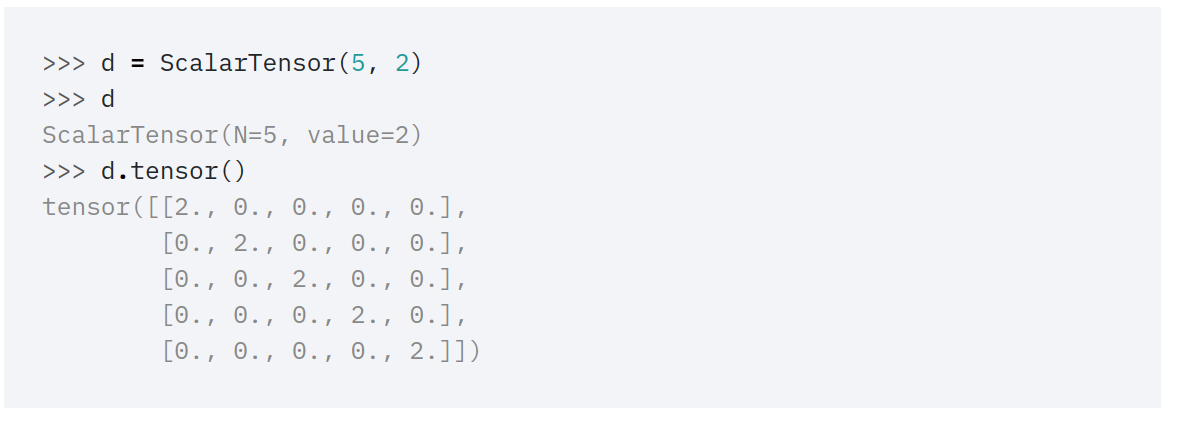If we try to use this object with the torch API, we will run into issues: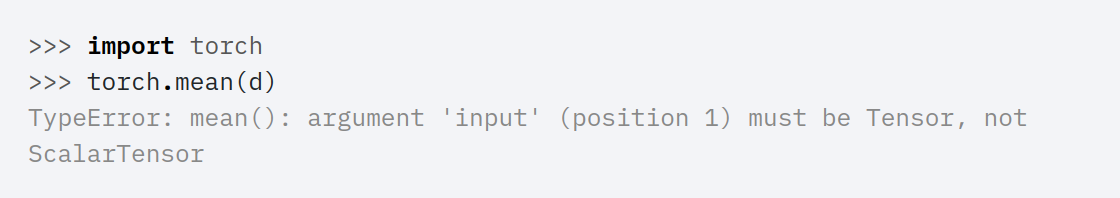Adding a __torch_function__ implementation to ScalarTensor makes it possible for the above operation to succeed. Let’s re-do our implementation, this time adding a __torch_function__ implementation:

HANDLED_FUNCTIONS = {}
class ScalarTensor(object):
def __init__(self, N, value):
self._N = N
self._value = value

def __repr__(self):
return "DiagonalTensor(N={}, value={})".format(self._N, self._value)

def tensor(self):
return self._value * torch.eye(self._N)

def __torch_function__(self, func, types, args=(), kwargs=None):
if kwargs is None:
kwargs = {}
if func not in HANDLED_FUNCTIONS or not all(
issubclass(t, (torch.Tensor, ScalarTensor))
for t in types
):
return NotImplemented
return HANDLED_FUNCTIONS[func](*args, **kwargs)


The __torch_function__ method takes four arguments:

• func, a reference to the torch API function that is being overridden,
• types, the list of types of Tensor-likes that implement __torch_function__,
• args, the tuple of arguments passed to the function, and
• kwargs, the dict of keyword arguments passed to the function.

It uses a global dispatch stable named HANDLED_FUNCTIONS to store custom implementations. The keys of this dictionary are functions in the torch namespace and the values are implementations for ScalarTensor.This class definition isn’t quite enough to make torch.mean do the right thing when we pass it a ScalarTensor – we also need to define an implementation for torch.mean for ScalarTensor operands and add the implementation to the HANDLED_FUNCTIONS dispatch table dictionary. One way of doing this is to define a decorator:

import functools
def implements(torch_function):
"""Register a torch function override for ScalarTensor"""
@functools.wraps(torch_function)
def decorator(func):
HANDLED_FUNCTIONS[torch_function] = func
return func
return decorator


which can be applied to the implementation of our override:

@implements(torch.mean)
def mean(input):
return float(input._value) / input._N


With this change we can now use torch.mean with ScalarTensor: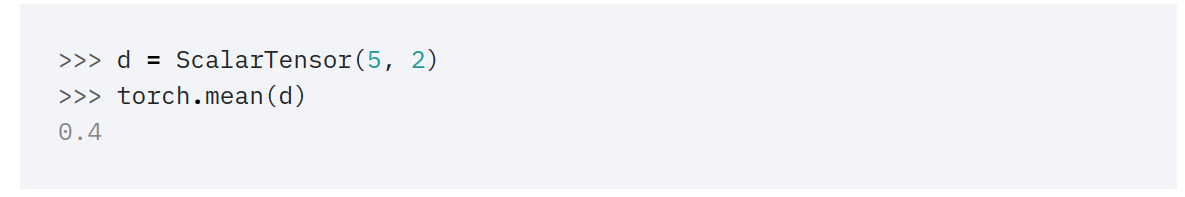Of course torch.mean is an example of the simplest kind of function to override since it only takes one operand. We can use the same machinery to override a function that takes more than one operand, any one of which might be a tensor or tensor-like that defines __torch_function__, for example for torch.add():

def ensure_tensor(data):
if isinstance(data, ScalarTensor):
return data.tensor()
return torch.as_tensor(data)

@implements(torch.add)
def add(input, other):
try:
if input._N == other._N:
return ScalarTensor(input._N, input._value + other._value)
else:
raise ValueError("Shape mismatch!")
except AttributeError:
return torch.add(ensure_tensor(input), ensure_tensor(other))


This version has a fast path for when both operands are ScalarTensor instances and also a slower path which degrades to converting the data to tensors when either operand is not a ScalarTensor. That makes the override function correctly when either operand is a ScalarTensor or a regular Tensor: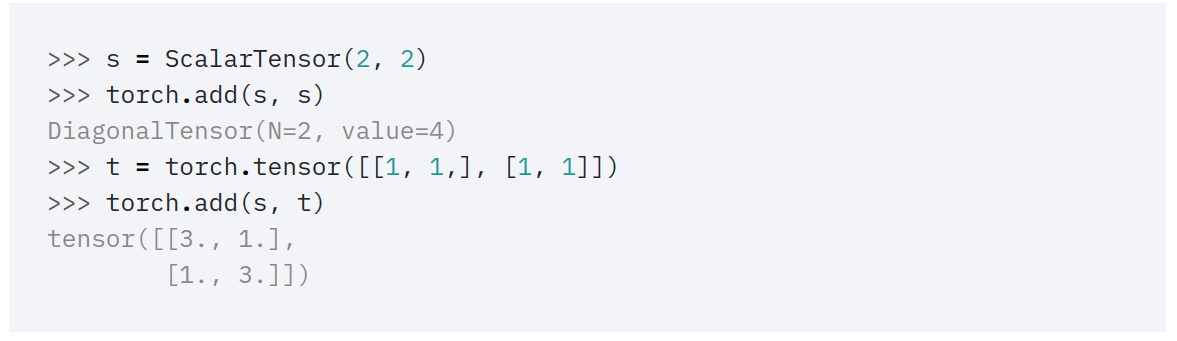Note that our implementation of add does not take alpha or out as keyword arguments like torch.add() does:

>>> torch.add(s, s, alpha=2)
TypeError: add() got an unexpected keyword argument 'alpha'


For speed and flexibility the __torch_function__ dispatch mechanism does not check that the signature of an override function matches the signature of the function being overridden in the torch API. For some applications ignoring optional arguments would be fine but to ensure full compatibility with Tensor, user implementations of torch API functions should take care to exactly emulate the API of the function that is being overridden.

Functions in the torch API that do not have explicit overrides will return NotImplemented from __torch_function__. If all operands with __torch_function__ defined on them return NotImplemented, PyTorch will raise a TypeError. This means that most of the time operations that do not have explicit overrides for a type will raise a TypeError when an instance of such a type is passed:

>>> torch.mul(s, 3)
TypeError: no implementation found for 'torch.mul' on types that
implement __torch_function__: [ScalarTensor]


In practice this means that if you would like to implement your overrides using a __torch_function__ implementation along these lines, you will need to explicitly implement the full torch API or the entire subset of the API that you care about for your use case. This may be a tall order as the full torch API is quite extensive.

Another option is to not return NotImplemented for operations that are not handled but to instead pass a Tensor to the original torch function when no override is available. For example, if we change our implementation of __torch_function__ for ScalarTensor to the one below:

def __torch_function__(self, func, types, args=(), kwargs=None):
if kwargs is None:
kwargs = {}
if func not in HANDLED_FUNCTIONS or not all(
issubclass(t, (torch.Tensor, ScalarTensor))
for t in types
):
args = [a.tensor() if hasattr(a, 'tensor') else a for a in args]
return func(*args, **kwargs)
return HANDLED_FUNCTIONS[func](*args, **kwargs)


Then torch.mul() will work correctly, although the return type will always be a Tensor rather than a ScalarTensor, even if both operands are ScalarTensor instances:

>>> s = ScalarTensor(2, 2)
>>> torch.mul(s, s)
tensor([[4., 0.],
[0., 4.]])


Also see the MetadataTensor example below for another variation on this pattern but instead always returns a MetadataTensor to propagate metadata through operations in the torch API.

### Extending torch with a Tensor wrapper type

Another useful case is a type that wraps a Tensor, either as an attribute or via subclassing. Below we implement a special case of this sort of type, a MetadataTensor that attaches a dictionary of metadata to a Tensor that is propagated through torch operations. Since this is a generic sort of wrapping for the full torch API, we do not need to individually implement each override so we can make the __torch_function__ implementation more permissive about what operations are allowed:

class MetadataTensor(object):
def __init__(self, data, metadata=None, **kwargs):
self._t = torch.as_tensor(data, **kwargs)
self._metadata = metadata

def __repr__(self):
return "Metadata:\n{}\n\ndata:\n{}".format(self._metadata, self._t)

def __torch_function__(self, func, types, args=(), kwargs=None):
if kwargs is None:
kwargs = {}
args = [a._t if hasattr(a, '_t') else a for a in args]
ret = func(*args, **kwargs)
return MetadataTensor(ret, metadata=self._metadata)


This simple implementation won’t necessarily work with every function in the torch API but it is good enough to capture most common operations:

>>> metadata = {'owner': 'Ministry of Silly Walks'}
>>> m = MetadataTensor([[1, 2], [3, 4]], metadata=metadata)
>>> t = torch.tensor([[1, 2], [1, 2]]])
>>> torch.add(t, m)
Metadata:
{'owner': 'Ministry of Silly Walks'}

data:
tensor([[2, 4],
[4, 6]])
>>> torch.mul(t, m)
Metadata:
{'owner': 'Ministry of Silly Walks'}

data:
tensor([[1, 4],
[3, 8]])


### Operations on multiple types that define __torch_function__

It is possible to use the torch API with multiple distinct types that each have a __torch_function__ implementation, but special care must be taken. In such a case the rules are:

• The dispatch operation gathers all distinct implementations of __torch_function__ for each operand and calls them in order: subclasses before superclasses, and otherwise left to right in the operator expression.

• If any value other than NotImplemented is returned, that value is returned as the result. Implementations can register that they do not implement an operation by returning NotImplemented.

• If all of the __torch_function__ implementations return NotImplemented, PyTorch raises a TypeError.

### Testing Coverage of Overrides for the PyTorch API

One troublesome aspect of implementing __torch_function__ is that if some operations do and others do not have overrides, users at best will see an inconsistent experience, or at worst will see errors raised at runtime when they use a function that does not have an override. To ease this process, PyTorch provides a developer-facing API for ensuring full support for __torch_function__ overrides. This API is private and may be subject to changes without warning in the future.

First, to get a listing of all overridable functions, use torch._overrides.get_overridable_functions. This returns a dictionary whose keys are namespaces in the PyTorch Python API and whose values are a list of functions in that namespace that can be overridden. For example, let’s print the names of the first 5 functions in torch.nn.functional that can be overridden:

>>> from torch._overrides import get_overridable_functions
>>> func_dict = get_overridable_functions()
>>> nn_funcs = func_dict[torch.nn.functional]
>>> print([f.__name__ for f in nn_funcs[:5])
['adaptive_avg_pool1d', 'adaptive_avg_pool2d', 'adaptive_avg_pool3d',
'adaptive_max_pool1d', 'adaptive_max_pool1d_with_indices']


This listing of functions makes it possible to iterate over all overridable functions, however in practice this is not enough to write tests for all of these functions without laboriously and manually copying the signature of each function for each test. To ease this process, the torch._overrides.get_testing_overrides function returns a dictionary mapping overridable functions in the PyTorch API to dummy lambda functions that have the same signature as the original function but unconditionally return -1. These functions are most useful to use with inspect to analyze the function signature of the original PyTorch function:

>>> import inspect
>>> from torch._overrides import get_testing_overrides
>>> override_dict = get_testing_overrides()
>>> dummy_add = override_dict[torch.add]
>>> inspect.signature(dummy_add)
<Signature (input, other, out=None)>


Finally, torch._overrides.get_ignored_functions returns a tuple of functions that explicitly cannot be overridden by __torch_function__. This list can be useful to confirm that a function that isn’t present in the dictionary returned by get_overridable_functions cannot be overridden.

## Writing custom C++ extensions

See this PyTorch tutorial for a detailed explanation and examples.

Documentations are available at torch.utils.cpp_extension.

## Writing custom C extensions

Example available at this GitHub repository.